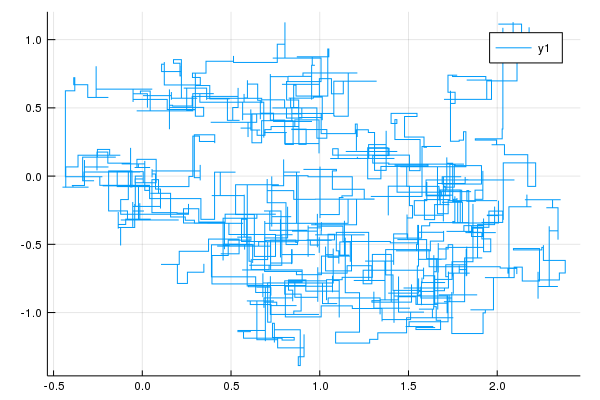## DynamicIterators.jl

Iterators with message passing and feedback loops
Author mschauer
Popularity
17 Stars
Updated Last
1 Year Ago
Started In
November 2018

# DynamicIterators.jl

## `DynamicIterator`

Iterators combine to a tree of iterators, but dynamic iterators combine to a network of interacting entities.

Dynamic iterators subtype `<:DynamicIterator`. They extend the iteration protocol and define

`    dyniterate(iter, somemessage(state))`

or

`    dyniterate(iter, othermessage(state), arg)`

where message wraps a state or other relevant information. For example the definition

```struct Start{T} <: Message
value::T
end
dyniterate(iter, Start(value))```

communicates that `iter` should start at `value` (if this is implemented). This is similar to `iterate(iter)` communicating that `iter` should start at a predefined value. In fact a fallback

`dyniterate(iter, ::Nothing) = iterate(iter)`

is in place.

Some messages make the iterator accept a third argument. A simple example using `bind` to bind an iterator to an iterator using the three-argument form of `dyniterate`:

```using DynamicIterators
import DynamicIterators: dyniterate

struct Summed <: DynamicIterator
end

function dyniterate(::Summed, ::Nothing, y)
y, y
end

function dyniterate(::Summed, i, y)
i + y, i + y
end

@show collect(bind(1:5, Summed()))```

A more in-depth example showing the power of the approach is https://github.com/mschauer/DynamicIterators.jl/blob/master/example/ressourcemanagement.jl, showing how to extend the iterator protocol to allow resource management (e.g. closing of files of child iterators) at the end of iteration of the parent.

A preliminary list of supported messages:

Message (and third argument) Meaning
`state` or `State(state)` ordinary iteration
`Start(noting)` start the iterator at its default
`Start(x)` start the iterate from the state corresponding to value `x`
`Value(x, state)` continue to iterate from the state corresponding to iterate `x`
`NextKey(state, nextkey)` advance an iterator over pairs of `key=>values` to `nextkey`
`Steps(state, n)` advance the iterator `n` steps or possibly rewind if `n` negative
`Control(state), control` control term as in the Kalman filter provided as third argument to dyniterate⋆
`Sample(state[,rng])` sample from iterates⋆
`NextKeys(state), key` advance iterator to the keys provided as third argument to dyniterate⋆

⋆persistent messages: `dyniterate` returns a state again wrapped by the message

## `Evolution`: Evolution-type dynamic iterators

Typically, the state of an iterator is opaque. But for some iterators the iterates are the states:

```julia> value, state = iterate('A':'Z')
('A', 'A')

julia> value, state = iterate('A':'Z', 'X')
('Y', 'Y')```

This means that the states/iterates of an iterator can be modified in a transparent way. This allows iterators not only to depend on each other, but to interact.

`DynamicIterators.jl` embeds a constrained iterator protocol for iterators subtyping `<:Evolution`, which define

```evolve(iterator, x) -> y
dub(x) = x === nothing ? nothing : (x,x)
iterate(iterator::Evolution, x) = dub(evolve(iterator, x))```

which guarantees `value == state` and introduces a powerful set of combinators for such iterators.

## Combinators

As a simple example take a Metropolis-Hastings chain

It can be described as a simple Evolution.

```function evolve(MH::MetropolisHastings, (t,x)::Pair)
P = MH.P
Q = MH.proposal(x)
xᵒ = rand(Q)
Qᵒ = MH.proposal(xᵒ)
if log(rand(MH.rng)) < MH.logpdf(P, xᵒ) - MH.logpdf(P, x) + MH.logpdf(Qᵒ, x) - MH.logpdf(Q, xᵒ)
x = xᵒ
end
(t+1 => x)
end```

The following example shows that the `Mixture` iterator combinator can be used to combine two Metropolis-Hastings chains into a component wise MetropolisHastings sampler:

```using DynamicIterators
using Distributions

D = MvNormal([1.0, 0.5], [1.0 0.5; 0.5 1.5] )
struct Move{T}
x::T
σ::Float64
i::Int
end
m1(x) = Move(x, 0.1, 1)
m2(x) = Move(x, 0.1, 2)
Base.rand(M::Move) = M.x + M.σ*randn()*[M.i-1, 2-M.i]
Distributions.logpdf(M::Move, x) = logpdf(Normal(M.x[M.i], M.σ), x[M.i])
MH1 = MetropolisHastings(D, m1, logpdf)
MH2 = MetropolisHastings(D, m2, logpdf)

I = Evolve(i->rand(1:2))

MH = mixture(I, (MH1, MH2))

X = values(trace(MH, 1=>(1, [0.0, 0.0]), endtime(2000)))```## Lifting time

Letting

`evolve(E, (i, x)::Pair) = i + 1 => evolve(E, x)`

constitutes a "lifting" of discrete time. This corresponds to enumerating the iterates of an evolution `x = f(x)` as `(1 => x1, 2 => x2, ...)`.

`DynamicIterators` control keywords treat `Pair`s as pair of key and value in concordance with the package `Trajectories` and somewhat in line with Julia's general convention.

## Examples

To illustrates the range of this I have picked some examples of very diverse nature.